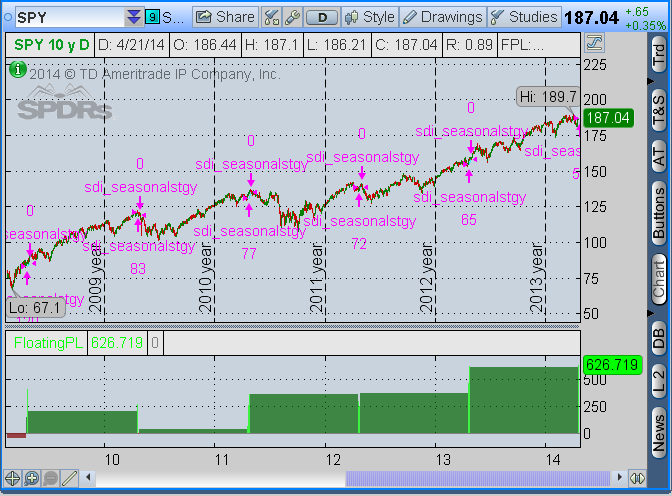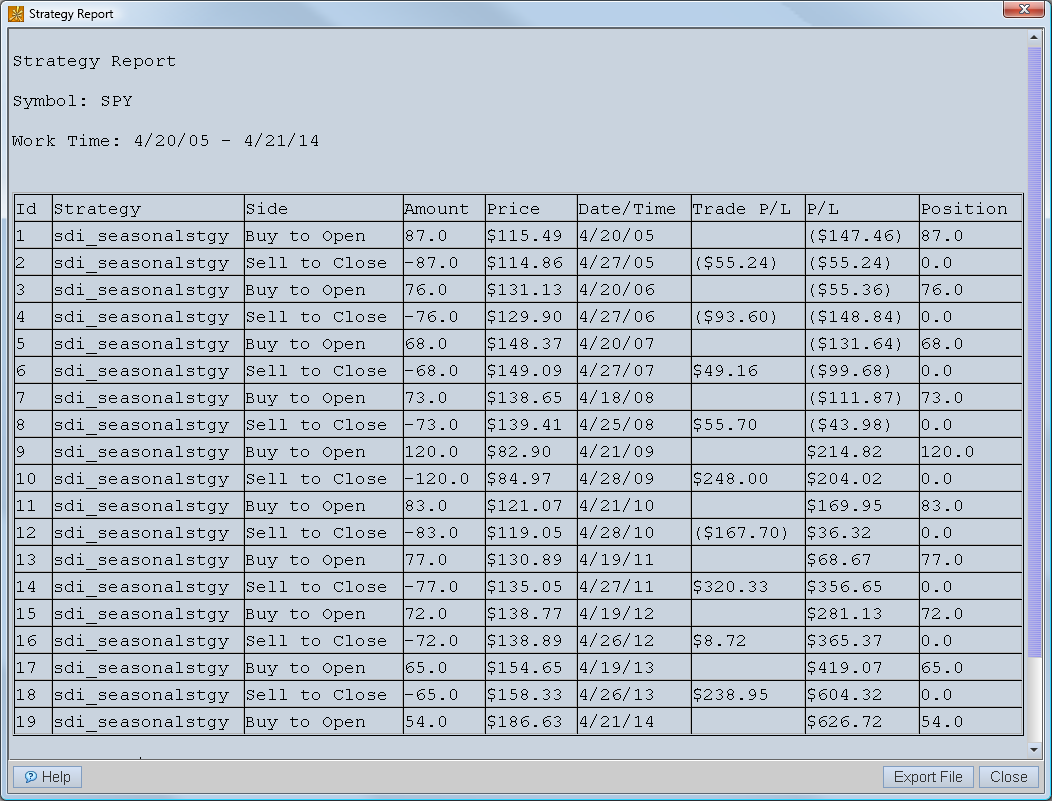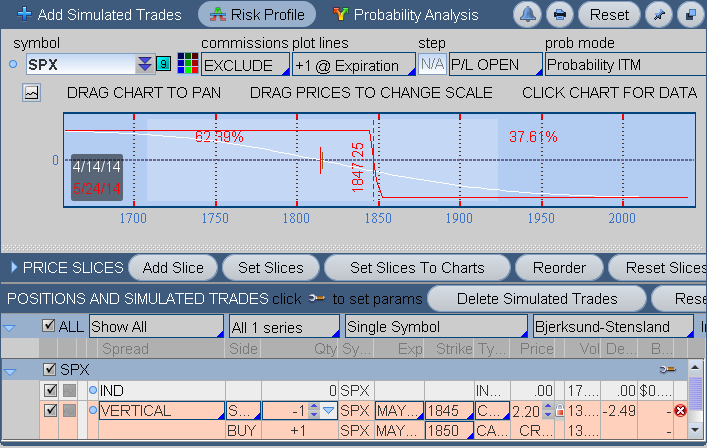## Wednesday, April 30, 2014

### thinkscript included: sdi_seasonalstgy rev: 1.0.1 released

previously sdi_seasonalstgy held the sim-trade 1 bar too long. this is corrected.

previously sdi_seasonalstgy would not initiate a sim-trade on first bar of the chart. it is typical that an n-year chart commences on the trigger bar and, because tos strategies always simulate trading on the next bar after a trigger, this meant that a potential data point was lost. now, if bar #1 is a trigger then sdi_seasonalstgy will add the sim-trade on bar #2 but with bar#1's closing price and the exit sim-trade will occur wrt bar#1.

previously sdi_seasonalstgy would not initiate a sim-trade before the first new-year on the chart. this resulted in a loss of a data point in an already small data-set. now sdi_seasonalstgy uses the countTradingDays function to calculate the trading day number of the year. most notably this will account for the missing trading days in some years such as 2012 when the market was closed for two days because of hurricane sandy. consequently, when the trading days corresponding to october 29 and 30 become the target of this strategy sim-trades for 2012 will not be initiated.

as always the updated revision is maintained on the original release page here.

## Monday, April 21, 2014

### thinkscript included: sdi_seasonalstgy - p&l on 10k invested seasonally and held short term

the seasonal strategy shows you the profit and loss of investing \$10,000 on the same trading day of the year as today going back up to 20 years. here's the image of this strategy at work:spy with sdi_seasonalstgy, shows the p&l of \$10k invested on the same trading day of the year and held for 10 trading days.
floatingPL, a standard tos chart study, shows the running total of the profit and loss of the strategy, which  purchases spy at the open on the same trading day of the year going back 10 years, the extent of the chart. 9 trades were taken resulting in a total profit of \$626.719 or about \$70 per trade, net, after commissions.

right clicking on the chart will drop down a menu with the show reports selection which, when clicked, produces the following:strategy report for seasonal purchases of spy showing 6 wins and 3 losses.

here's the thinkscript:

## Sunday, April 20, 2014

### how to tell if your vertical debit spread is in the kelly zone

in a vertical debit spread you are paying upfront for a combination of long and short options. to tell if the price you are paying for the spread falls into the positive kelly zone the risk you pay upfront for the reward must be low enough. here is the the formula i use to determine where this even-money price point is:
e = p * w
• e is the even-money price
• p is the probability of success
• w is the width of the strikes
for example, if you are pricing a put vertical on spy that has a width of \$1 between the long and short options and the tos analyze tab shows a probability of success of  54% then the  price one should pay to be at even-money is 54 cents. if you can get it cheaper then you are in the kelly-zone, where the kelly formula will give a positive number.

## Tuesday, April 15, 2014

### how to tell if your vertical credit spread is in the kelly zone

the criterion part of the kelly criterion is that the candidate trade must have an edge. the wiki page says that for the kelly number to be positive the following must be true:
b > q/p
• b is the benefit to risk ratio
• q is the probability of loss
• p is the probability of success
applying the kelly criterion to vertical credit spreads results in a simple pricing rule by which one may calculate the even-money price for the spread. here 'tis:
c = q * w
• c is the even-money credit collected for the vertical.
• q is the probability of loss, as above.
• w is the price width between the strikes.
for example, if you are pricing a put vertical on spy that has a width of \$1 between the long and short options and the tos analyze tab shows a probability of loss of 37% then the credit one must collect to be at even-money is 37 cents. if you can collect more than that then you are in the kelly-zone, where the kelly formula will give a positive number.

## Monday, April 14, 2014

### a 30-delta, kelly-positive credit spread on spx

i was expecting high probability credit spreads that are kelly-positive to be as rare as unicorns, so i was surprised to find one in the spx at a 30 delta strike but here 'tis:bear call spread at 38 delta expiring in 40 days in may4 expiration
the benefit-to-risk, b, is .7857 - this 30 delta spread is pricing at nearly 50% of the strike width. the probability of success, p, is .6239. this gives a kelly number of: 0.145 (=(.6239*1.7857-1)/.7857)

this implies a huge edge in this trade. if you recall my previous post that jeff ma had a mathematical edge in blackjack of just 2% from the science of card-counting and that this translated into a .02 kelly number.  well .14 is 7 times bigger.  what is going on?

there may be some speculation on a buy-the-dip event, possibly bear traders buying upside calls for protection.

well ... maybe.

## Sunday, April 13, 2014

here is the rule of thumb i was taught for finding credit spreads - look for a 30'ish delta option expiring in 40 days or so - then sell that option and buy the next strike further out-of-the-money. on the spx i find trades like this:spx put credit spread at 37 delta, expiring in 40 days
here's what kelly has to say about this trade: our p is .6066 and b is 1.45/3.55=.408, thus the kelly number is -0.35 =((.6066*1.408-1)/.408). so if you have been taking trades like this and your net liq is net licked then maybe this is the reason ... or not.

1. how much credit do you need to collect on a credit spread to make kelly happy?
2. how common are kelly-positive credit spreads?
4. does a time-stop change the story?
5. does an early buyback change the story?
6. does a sense of direction change the story?

﻿

## Saturday, April 12, 2014

### the kelly number

the kelly criterion is a number between -1 and +1 that tells you the maximum percentage of your account to allocate to a defined risk trade in order to have positive expectancy without blowing out your account. a positive kelly number is a green-light, a negative kelly number says that the edge lies with the opposite side of the trade. by positive expectancy i mean that there is a theoretical chance of gain, over time, once all the wins and losses have been totalled.

here is the formula:
f = (p * (b+1)-1)/b
• f is the kelly number
• p is the probability of success
• b is the return/risk ratio (sometimes called the odds.)
so consider the fate of coin flippers. p is obviously .5 and in the typical example b is 1 i.e. risk 1 to make 1. in this situation the kelly number, f, is 0 (=(.5*(1+1)-1)/1). this is a mistake for either side to play this game.

now suppose you are jeff ma (the real-life protagonist of the movie 21, the big-player for the infamous mit blackjack team, and the author of the house advantage in my brane fud section) and you sit down at a blackjack table for which your confederate has signalled that the count favors players 51:49. how much do you bet? the kelly number is .02 (=.51*2-1). if you have 50K in your bankroll then you bet \$1000 a hand.

next i apply kelly to vertical-option spreads ...